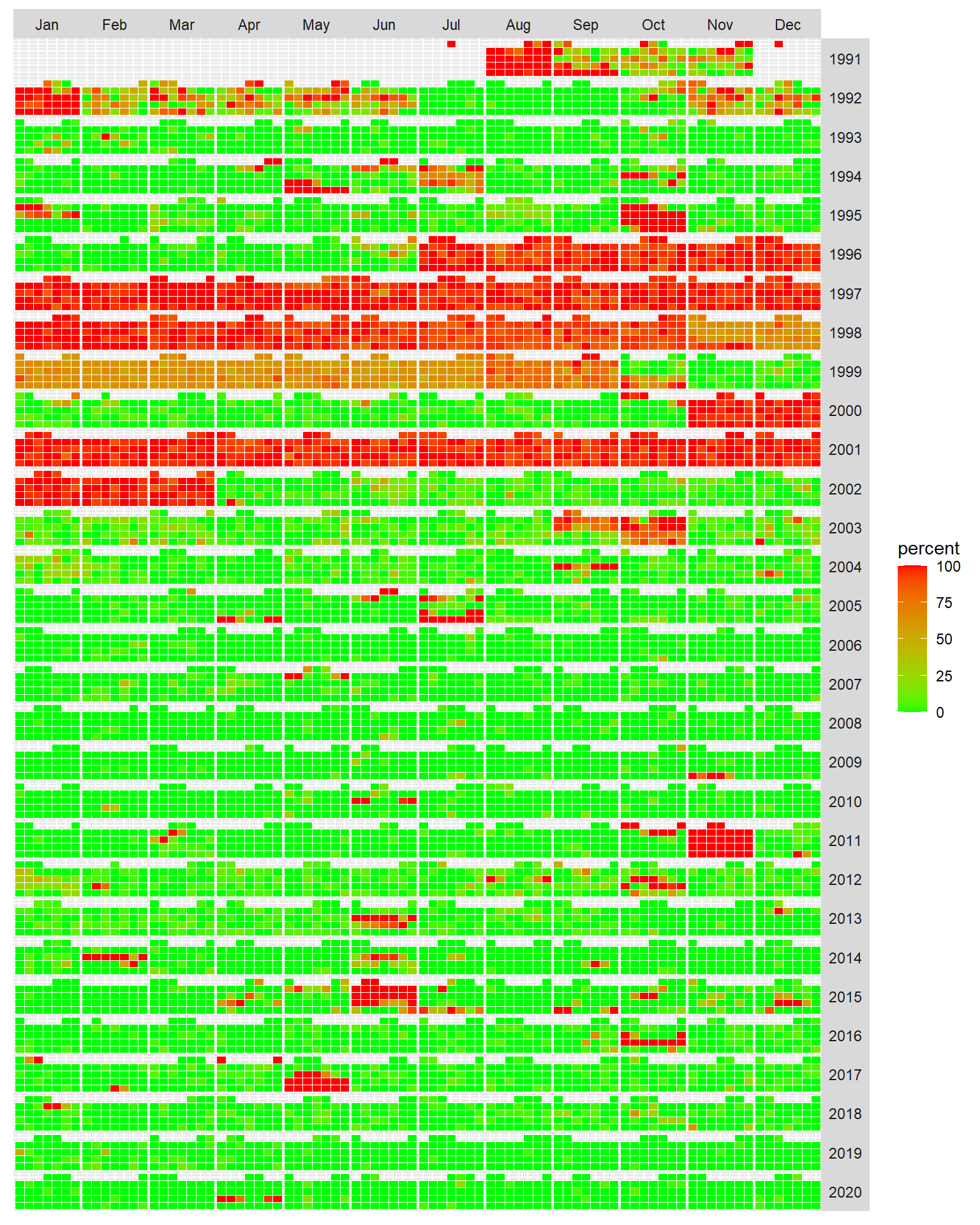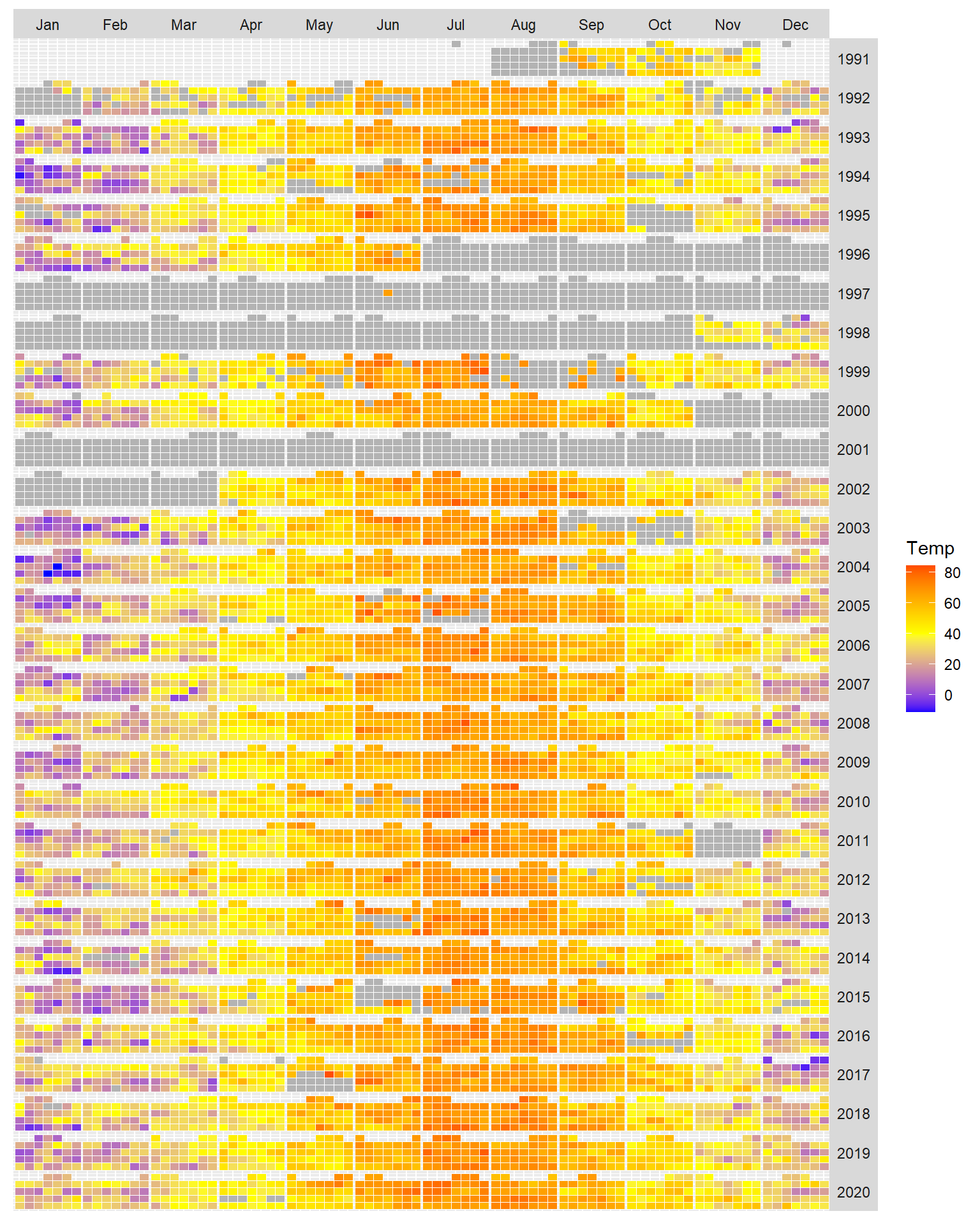The following are examples of exploratory plots generated from meteorological data for Waterville, Maine (USA). Data were pulled from ftp://ftp.ncdc.noaa.gov/pub/data/noaa/isd-lite/ then saved in a native R file format named `met1991_2020.Rds` available on this repo using the `Meteo_lite.R` script (also available on this repo).

# Data summary

The dataset spans the years 1991 through 2020. A data summary follows.

``````library(MASS)
library(dplyr)
library(lubridate)
library(ggplot2)

stargazer::stargazer(as.data.frame(dat), type = "html", flip = FALSE,
title="Descriptive statistics", digits = 2,
mean.sd = FALSE, no.space = FALSE )``````
 Statistic N Mean St. Dev. Min Pctl(25) Pctl(75) Max Temp 202,654 45.11 19.72 -22.00 30.20 60.80 98.60 DP 201,817 34.11 20.74 -32.80 19.40 51.80 80.60 Speed 202,668 5.79 4.76 0.00 3.36 8.05 40.26 Dir 197,247 162.41 131.94 0.00 10.00 290.00 360.00 Press 42,867 1,018.18 9.12 974.20 1,012.60 1,024.20 1,048.20 Precip_1hr 40,941 0.02 0.21 -0.004 0.00 0.01 10.00 Precip_6hr 7,221 0.11 0.45 -0.004 0.00 0.09 10.00

# Temperature exploratory analysis

## Plot of percent missing values

With a large temporal dataset, it’s always a good idea to scan for missing values. The following plot is a calendar heatmap showing the percentage of missing temperature values. Each block element represents a day.

``````dat %>% mutate(missing = as.numeric(is.na(Temp)),
month = month(Date_EST, label=TRUE,abbr=TRUE),
year  = year(Date_EST),
weekday = wday(Date_EST,label=TRUE),
monthw = ceiling(day(Date_EST) / 7) ) %>%
group_by(month,year,weekday,monthw) %>%
summarise(percent = mean(missing) * 100) %>%
ggplot() + aes( weekday, monthw,fill = percent) +
geom_tile(colour = "white") +
facet_grid(year~month) +
theme(axis.title=element_blank(),
axis.text=element_blank(),
axis.ticks=element_blank(),
strip.text=element_text(angle=0),
strip.text.y=element_text(angle=0),
panel.spacing = unit(0, "lines"))``````There’s a large swath of missing values for the years 1996 through 1999 and for the year 2001.

## Heatmap of temperature data

Next, we’ll generate a median temperature heatmap (in degrees Fahrenheit) but limit the output to days with fewer than 70% missing values. Days for which more than 70% of values are missing are assigned a grey color.

``````dat %>% mutate(missing = as.numeric(is.na(Temp)),
month = month(Date_EST, label=TRUE,abbr=TRUE),
year  = year(Date_EST),
weekday = wday(Date_EST,label=TRUE),
monthw = ceiling(day(Date_EST) / 7) ) %>%
group_by(month,year,weekday,monthw) %>%
summarise(percent = mean(missing) * 100,
Temp = ifelse(percent > 70, NA, median(Temp, na.rm=TRUE))) %>%
ggplot() + aes( weekday,monthw, fill = Temp) +
geom_tile(colour = "white") +
facet_grid(year~month) +
na.value = "grey70", midpoint = 40) +
theme(axis.title=element_blank(),
axis.text=element_blank(),
axis.ticks=element_blank(),
strip.text.y=element_text(angle=0),
panel.spacing = unit(0, "lines"))``````## Heatmap of smoothed temperature data

The following heatmap applies a loess smooth surface to the data. This technique helps visualize overall patterns in the two dimensional dataset. As small loess span (~ `0.04`) is adopted to help capture small (time) scale variations on the data. To avoid edge effects at the beginning and end of each year, the data are wrapped when fitting a loess surface. But wrapping is not applied to the ends of the date ranges (i.e. prior to 2003 and after 2020). Note that because of the large swaths of missing values, data are restricted to 2003 and up.

``````dat.loess <- dat %>%
select(Temp, Date_EST) %>%
na.omit() %>%
filter(year(Date_EST) > 2002) %>%
mutate(date = as.Date(Date_EST)) %>%
group_by(date) %>%
summarise(temp = mean(Temp, na.rm = TRUE)) %>%
ungroup() %>%
mutate(dec.year = decimal_date(date),
year = floor(dec.year),
day= yday(date)) %>%
select(-dec.year)

# Wrap dates to correct for temporal edge effects
dat.loess.pre <- dat.loess %>%
filter(day > 250) %>%
mutate(year = year + 1,
day = day - 366)

dat.loess.post <- dat.loess %>%
filter(day < 100) %>%
mutate(year = year - 1,
day = day + 366)

dat.lowess.wrap <- rbind(dat.loess, dat.loess.pre, dat.loess.post)

dat.smooth <- loess(temp ~ year + day, dat.lowess.wrap,
span = 0.04, degree  = 2,
family = "symmetric")

smooth.df <- data.frame(dat.smooth\$x, dat.smooth\$fitted) %>%
filter(day > 0 & day < 366)

ggplot(smooth.df) + aes( day , year,
fill = `dat.smooth.fitted`,
z = `dat.smooth.fitted`) +
geom_raster(interpolate = TRUE) +